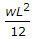# Civil Engineering - Theory of Structures

### Exercise :: Theory of Structures - Section 3

26.

A steel plate d x b is sandwiched rigidly between two timber joists each D x B/2 in section. The moment of resistance of the beam for the same maximum permissible stress σ in timber and steel will be (where Young's modulus of steel is m times that of the timber).

 A.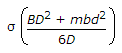B.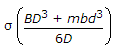C.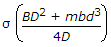D.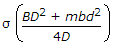Explanation:

No answer description available for this question. Let us discuss.

27.

The ratio of crippling loads of a column having both the ends fixed to the column having both the ends hinged, is

 A. 1 B. 2 C. 3 D. 4

Explanation:

No answer description available for this question. Let us discuss.

28.

Maximum strain theory for the failure of a material at the elastic limit, is known as

 A. Guest's or Trecas' theory B. St. Venant's theory C. Rankine's theory D. Haig's theory E. Von Mises's theory.

Explanation:

No answer description available for this question. Let us discuss.

29.

A cantilever of length L is subjected to a bending moment M at its free end. If EI is the flexural rigidity of the section, the deflection of the free end, is

 A.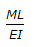B.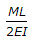C.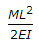D.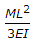Explanation:

No answer description available for this question. Let us discuss.

30.

The maximum bending moment for a simply supported beam with a uniformly distributed load w/unit length, is

 A.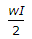B.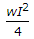C.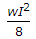D.